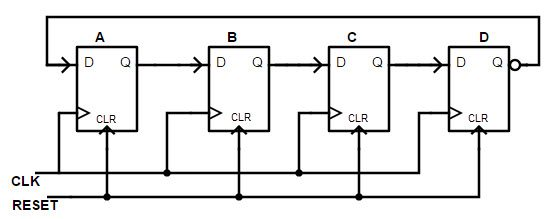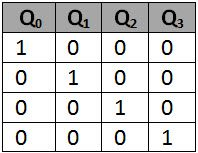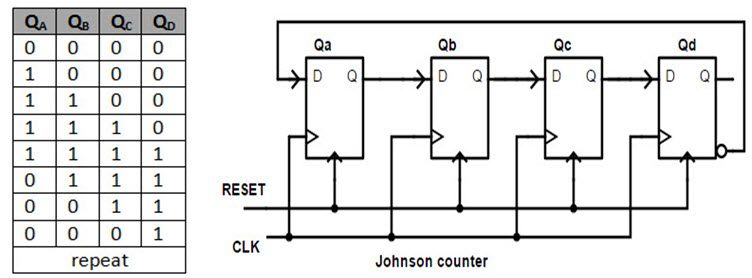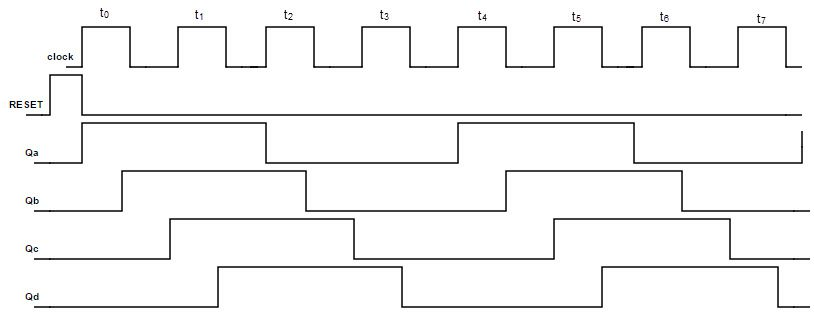## Home# Ring counters (Johnson Ring Counter)

Ring counter is a sequential logic circuit that is constructed using shift register. Same data recirculates in the counter depending on the clock pulse.

Ring counters are of two types

1)Ordinary Ring counters

2) Johnson counter

Outline

### 4 bit Ring Counter

The ring counter is a cascaded connection of flip flops, in which the output of last flip flop is connected to input of first flip flop. In ring counter if the output of any stage is 1, then its reminder is 0. The Ring counters transfers the same output throughout the circuit.

That means if the output of the first flip flop is 1, then this is transferred to its next stage i.e. 2nd flip flop. By transferring the output to its next stage, the output of first flip flop becomes 0. And this process continues for all the stages of a ring counter. If we use n flip flops in the ring counter, the ‘1’ is circulated for every n clock cycles.

The circuit diagram of the ring counter is shown below.Here we design the ring counter by using D flip flop. This is a Mod 4 ring counter which has 4 D flip flops connected in series. The clock signal is applied to clock input of each flip flop, simultaneously and the RESET pulse is applied to the CLR inputs of all the flip flops.

#### Operation of Ring Counter

Initially, all the flip flops in ring counter are reset to 0 by applying CLEAR signal. Before applying the clock pulse, we apply the PRESET pulse to the flip flops which assigns the value ‘1’ to the ring counter circuit. For each clock signal, the data circulates among all the 4 flip flop stages of ring counter.

This 4 staged ring counter is called Mod 4 ring counter or 4 bit ring counter. To circulate the data correctly in the ring counter, we must load the counter with required values like all 0’s or all 1’s.

##### Circulation of data in Ring countersWe know that the ring counter is similar to that of the shift registers connected in series. The above diagram shown the four stages of flip flops as the parallel in serial our shift registers, with data inputs D0, D1, D2 and D3.

The data circulation in ring counter is explained below. By passing the reset signal, initially the flip flops are at RESET state. When the PRESET is applied to the ring counter the input of the circuit becomes 1.

This input is connected to the first flip flop in the series, so that the flip flop QA is set to 1 and all other outputs of remaining flip flops will be low.

If we make the data input of the flip flop ‘A’ to low, this gives us the data pulse as 0 1 0. Then for the second clock signal, the output of first flip flop will again change and then the output of ‘B’ will become high. This means the data pulse 0 0 1 occurs.

In this way, as the clock signal and input of first flip flop changes, the output of the other flip flops changes. As the output of last flip flop in series is connected to the input of the first flip flop, the data sequence rotates or circulates in the ring counter.

#### Truth table of ring counter

The truth table of the 4 bit ring counter is explained below.When CLEAR input CLR = 0, then all flip flops are set to 1. When CLEAR input CLR = 1, the ring counter starts its operation. For one clock signal, the counter starts its operation. On next clock signal, the counter again resets to 0000. Ring counter has 4 sequences: 0001, 0010, 0100, 1000, 000.

#### Timing diagram of Ring CounterThe timing diagram of the Ring counter will explain that the clock signal changes the output of every stage of the counter, so that CLK signal will help the data to circulate from one flip flop to another. As the 4 bit ring counter (4 stages or 4 flip flops) circulates the preset digit within one clock signal, the output frequency of each flip flop is ¼ th of the main clock frequency.

#### State diagram of ring counterThe state diagram of the 4 bit ring counter is shown in above picture. It denotes that the position of the preset digit (in this case preset digit is 1) is changing its position from LSB to MSB, for one clock signal.

• Can be implemented using D and JK flip-flops. It is a self-decoding circuit.

• Only four of the 15 states are being utilized.

### Johnson Counter

The Johnson counter is a modification of ring counter. In this the inverted output of the last stage flip flop is connected to the input of first flip flop. If we use n flip flops to design the Johnson counter, it is known as 2n bit Johnson counter or Mod 2n Johnson counter.

This is an advantage of the Johnson counter that it requires only half number of flip flops that of a ring counter uses, to design the same Mod.

The main difference between the 4 bit ring counter and the Johnson counter is that , in ring counter , we connect the output of last flip flop directly to the input of first flip flop. But in Johnson counter, we connect the inverted output of last stage to the first stage input.

The Johnson counter is also known as Twisted Ring Counter, with a feedback. In Johnson counter the input of the first flip flop is connected from the inverted output of the last flip flop.

The Johnson counter or switch trail ring counter is designed in such a way that it overcomes the limitations of ring counter. Mainly it reduces the number of flip flops required for designing the circuit.Similar to the ring counter, the clock signal in johnson counter is connected to the clock input of each flip flop simultaneously.

#### Operation of johnson counter

The Johnson counter designed with D flip flop is shown below. It has four stages i.e. four flip flops connected in series type or cascaded. Initially zero / Null is fed to the Johnson counter and on applying the clock signal, outputs will change to “1000”, “1100”, “1110”, “1111”, “0111”, “0011”, “0001”, “0000” in a sequence and the sequence will repeat for next clock signal.

The Johnson counter produces a special pattern by passing four 0’s and then four 1’s and thus it produces a special pattern by counting up down.

#### Truth table of johnson counter

The truth table of the 4 bit ring counter is explained below.The state diagram indicates that how the data transfers from one flip flop to another for every clock pulse. The4 stage Johnson ring counters are used as frequency dividers, by varying their feedback connections. So they can be used as frequency divider circuits also.

#### Timing diagram of johnson counterThe timing diagram of the johnson counter will explain that the clock signal changes the output of every stage of the counter, so that CLK signal will help the data to circulate from one flip flop to another.When CLR = 0, all outputs and inputs of flip flops are preset to 0 (cleared) except the data input of right most FF which sets to 1.

When CLR = 1, Johnson counter starts its operation. On every clock edge, the output of last flip flop (1) shifts left to the third flip flop. As the first flip flop is connected to serial input i.e. 1, the input of third flip flop is 1.

In next cycle, QA = 0 so 0 rotates in ring form in second half cycle. Johnson counter has 8 sequences: 0001, 0011, 0111, 1111, 1110, 1100, 1000, and 0000.

Advantage of Johnson counter is that, it has more outputs than ring counter.

Disadvantage of Johnson counter is that , Only out of 15 states are only 8 are used.

### Applications of Ring counters

• Ring counters are used to count the data in a continuous loop.
• They are also used to detect the various numbers values or various patterns within a set of information, by connecting AND & OR logic gates to the ring counter circuits.
• 2 stage, 3 stage and 4 stage ring counters are used in frequency divider circuits as divide by 2 and divide by 3 and divide by 4 circuits, respectively.
• The 3 stage Johnson counter is used as a 3 phase square wave generator which produces 1200 phase shift.
• The 5 stage Johnson counter circuit is generally used as synchronous decade (BCD) counter and also as divider circuit.
• The 2 stage Johnson counters are also known as “Quadrature oscillator” which is used to produce 4 level individual outputs which are out of phase with 900 with each other. This quadrature generator is used to produce 4 phase timing signal.

### 2 Responses

1.Rekha says:

Nice explanations icompletetly understood it but i want about 5-bit johnson ring counter

2.manjeet says:

Sir how to make 5 bit ring counter